How To Establish Sample Sizes For Process Validation Using Statistical Tolerance Intervals

By Mark Durivage, Quality Systems Compliance LLC

The first article in this series, Risk-Based Approaches To Establishing Sample Sizes For Process Validation (June 2016), established the relationship between risk and sample size. Subsequent articles have introduced different methods for determining sample sizes to ensure validation activities will yield valid results. This installment will demonstrate how to use statistical tolerance limits, which use the confidence level (how sure we are) and reliability value (population value) to determine appropriate statistically valid sample sizes for process validation.

Before we begin, we must establish our definitions of risk and their associated confidence level and reliability value. These definitions can and should vary based upon the organizational needs.  A good place to determine the risk level is failure mode and effects analysis (FMEA), a systematic group of activities designed to recognize, document, and evaluate the potential failure of a product or process and its effects. FMEA uses a risk priority number (RPN), which is based on the frequency, detection, and severity of a potential failure mode. The higher the RPN, the higher the risk. However, a low probability of occurrence in conjunction with high severity and high probability of detection may still necessitate the appropriate controls for high risk.

Table 1 depicts an example FMEA with the associated risk levels.  Once the risk level has been determined (low, medium, high), the appropriate confidence level and reliability can be selected using Table 3. Figure 1 depicts the linkage between FMEA, risk, and confidence level and reliability.

Table 1: Example FMEA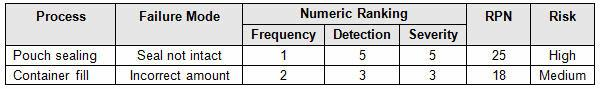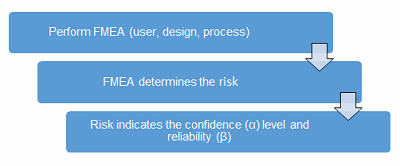Figure 1: Risk process for determining the appropriate confidence level and reliability

Table 2 shows an example of risk level definitions with accompanying defect classifications. These definitions can and will vary based upon the product(s) produced and its intended and unintended uses.

Table 2: Example of Risk Level Definitions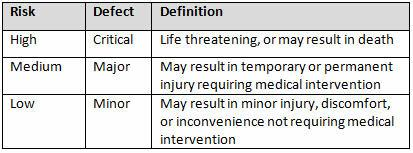Table 3 depicts example confidence levels and reliability values based upon risk. Of course, different confidence and reliability levels can and should be utilized based upon an organization’s risk acceptance determination threshold, industry practice, guidance documents, and regulatory requirements.

Table 3: Example Confidence and Reliability Levels Based upon Risk Acceptance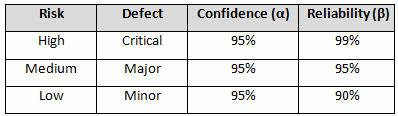Statistical Tolerance Intervals

When using statistical tolerance intervals, there is an assumption and expectation that the data is normally distributed. There are many ways to determine if data is normally distributed, including computer programs and spreadsheets. However, for small samples (15 or fewer) normal probability plots can be used to assess normality.

Normal probability plots can be constructed to look for linearity when using one variable. Normal probability plots provide a visual way to determine if a distribution is approximately normal. If the distribution is close to normal, the plotted points will lie close to a line.  Normal probability plots are constructed by doing the following:

1. Arrange the data from smallest to largest.
2. Determine the percentile of each data value.
3. From these percentiles, do normal calculations to determine their corresponding z-scores.
4. Plot each z-score against its corresponding data value.
5. Perform a graphical review of the normal probability plot for the data points to verify if they generally fall on the line of best fit — sometimes referred to as the “pencil” test. If the data is not normally distributed, it is best to use an alternative method.

(For an example of how to create and analyze normal probability plots, refer to Chapter 4 of Practical Engineering, Process, and Reliability Statistics, ASQ Quality Press, 2014.)

Statistical tolerance intervals can be one-sided or two-sided, depending if the specification requires a minimum or maximum value or if the specification has an allowable range. However, it is generally best practice to use one-sided tolerance intervals — this method will provide a more conservative approach, because the risk is placed on one side rather than being split. If the specification is bilateral, use the specification that is closest to the sample mean, which is calculated from the initial sample.

The first step in using statistical tolerance intervals to determine sample sizes for process validation is to calculate the mean and standard deviation from a small sample, which should capture the expected range of variation that can reasonably be expected from the process (different batches of materials, operators, etc.). To determine this minimum initial sample for a one-sided test: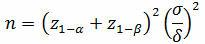And for a two-sided test: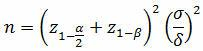Where:

z = normal distribution value

α = confidence

β = reliability

δ = the difference or shift we want to detect

Table 4 provides the number of samples required to detect either a 1.0σ shift or a 1.5σ shift. If the testing to be performed is destructive, expensive, or on high-value parts, using a 1.5σ shift is suggested; otherwise use a 1.0σ shift. Please note that these are the minimum number of samples to establish the sample mean and standard deviation.

Table 4: Initial Sample Table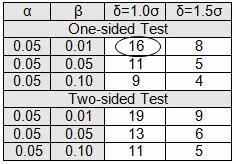Single-Sided Specification Example

A pouch-sealing operation is considered high risk according the FMEA in Table 1. High risk requires 95% confidence (0.05α) and 99% reliability (0.01β), as shown in Table 3. The validation team decided to use a δ of 1.0σ. Table 4 requires an initial sample of 16. The 16 samples were found to be normally distributed, with a mean of 3.2 lbs and sigma of 0.23. The pouch-sealing process specification requires a minimum pull strength of 2.5 lbs.

For a single-sided specification: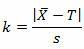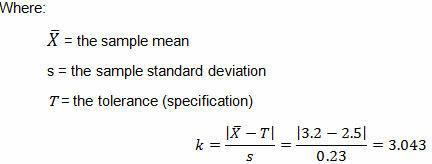Table 5: 95% One-Sided Tolerance k Factors (Partial)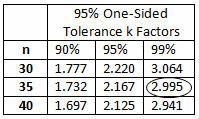The calculated k value is 3.043. (The k value selected should be equal to or less than the calculated value.) High risk requires 95% confidence (0.05α) and 99% reliability (0.01β), for which Table 5 indicates a minimum of 35 samples. A minimum of 35 samples must be used for the validation. Because the company typically uses three batches for process validation, 12 samples will be randomly drawn from each of three batches for a total of 36 samples.

Three validation lots were manufactured. The 36 samples were found to be normally distributed with a mean of 3.1 lbs and sigma of 0.21.

For a one-sided tolerance: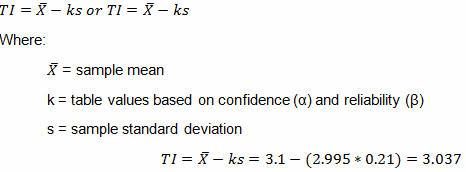Since the calculated tolerance interval (3.037 lbs) is greater than the minimum pull strength of 2.5 lbs, the validation passed. We can state that we are 95% confident that the process is 99% reliable. In other words, we can claim that 95% of the time, 99% of the products produced will exceed the minimum pull strength of 2.5 lbs.Sample size does matter! Let Mark Durivage show you which method for determining sample size is best for your process validation efforts:

Risk-Based Approaches To Establishing Sample Sizes For Process Validation

February 16, 2017 | 1:00-2:30PM EST

Double-Sided Specification Example

A filling operation is considered to be medium risk according the FMEA in Table 1. Medium risk requires 95% confidence (0.05α) and 95% reliability (0.05β), as shown in Table 3. The validation team decided to use a δ of 1.0σ. Table 4 requires an initial sample of 13. The 13 samples were found to be normally distributed, with a mean 995 g and sigma of 4.3. Filling process specification is 1000 g ±5 g.

For a double-sided specification: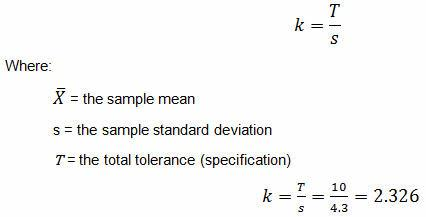The calculated k value is 2.326. (The k value selected should be equal to or less than the calculated value.) Medium risk requires 95% confidence (0.05α) and 95% reliability (0.05β), which Table 6 indicates a minimum of 80 samples. A minimum of 80 samples must be used for the validation. Because the company typically uses three batches for process validation, 27 samples will be randomly drawn from each of three batches for a total of 81 samples.

Three validation lots were manufactured. The 81 samples were found to be normally with a sample mean of 994 g and sample standard deviation of 4.2.

Table 6: 95% Two-Sided Tolerance k Factors (Partial)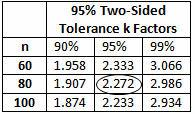For a two-sided tolerance: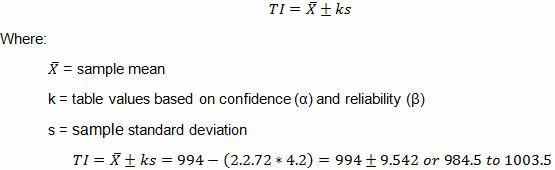Recall the tolerance is 1000 g ±5 g (995 g to 1005 g). Since the lower tolerance interval (984.5 g) is below the lower specification of 995 g, the validation has failed. It should be apparent that there are two issues with the process. The first is that the process is not centered, and second is that there is excessive variation. The validation team must first center the process and then determine the best method(s) to reduce the variation within the process.

Choosing The Right Method For Your Process

Although the examples provided use single-sided and double-sided specifications, in practice I prefer to use the single-sided method, to account for an off-center process (same principle as Cpk). Remember, the criteria used should be based upon risk and should be documented and proceduralized. When a validation fails, there are three distinct options available:

• Center the process
• Reduce the variation
• Change the specification (tolerance)

I want to reinforce that different confidence levels and reliability values can and should be utilized based upon an organization’s risk acceptance determination threshold, industry practice, guidance documents, and regulatory requirements. Using statistical tolerance intervals to establish sample sizes for process validation activities is a widely used and accepted practice in FDA-regulated industries.

The methods presented here have been used and successfully defended during audits and inspections. I cannot emphasize enough the importance of proceduralizing (documenting) the statistical methods and rationale your organization may use for process validation activities. Table 2 provides an example of documenting and standardizing risk levels, defect classifications, and defect definitions. Table 3 provides an example for documenting the confidence level and reliability requirements for process validation activities. I also recommend that validation and statistical technique procedures include the formulas as well as fully worked examples, like those demonstrated above, to provide clarity and guidance for those individuals writing, performing, executing, and approving process validation activities.

Subsequent articles in this series will provide additional how-to examples for applying risk-based sample size techniques to process validations in your organization. Previous articles include:Mark Allen Durivage is the managing principal consultant at Quality Systems Compliance LLC and an author of several quality-related books. He earned a B.A.S in computer aided machining from Siena Heights University and an MS in quality management from Eastern Michigan University. Durivage is an ASQ Fellow and holds several ASQ certifications including CQM/OE, CRE, CQE, CQA, CHA, CBA, CPGP, and CSSBB. He also is a Certified Tissue Bank Specialist (CTBS) and holds a Global Regulatory Affairs Certification (RAC). Durivage resides in Lambertville, Michigan. Please feel free to email him at mark.durivage@qscompliance.com with any questions or comments, or connect with him on LinkedIn.